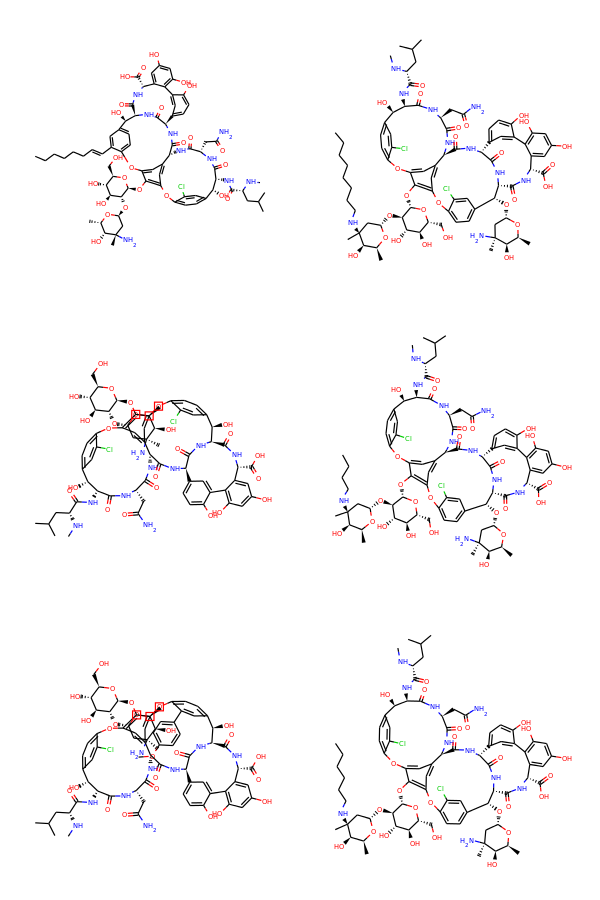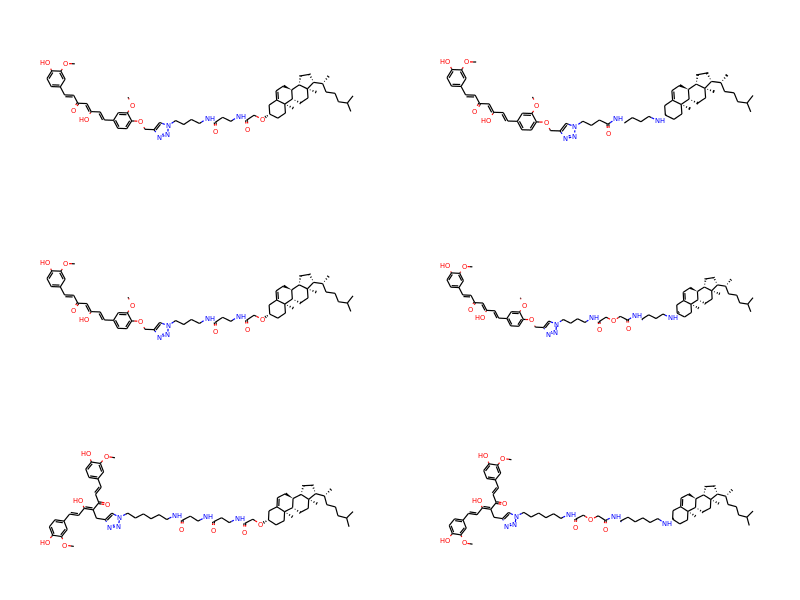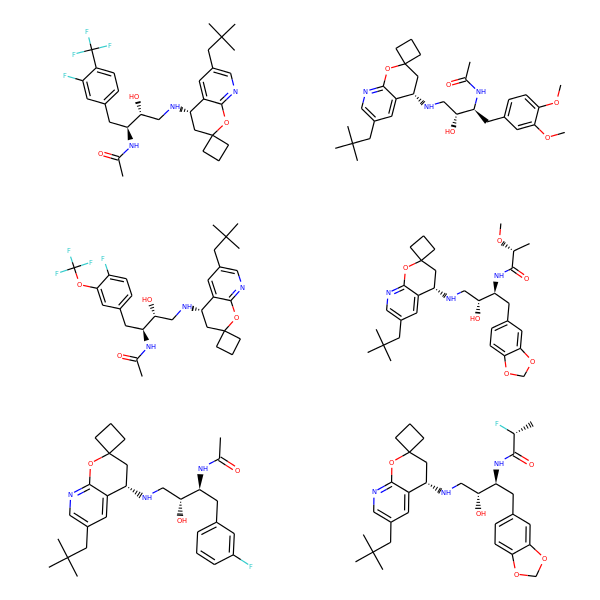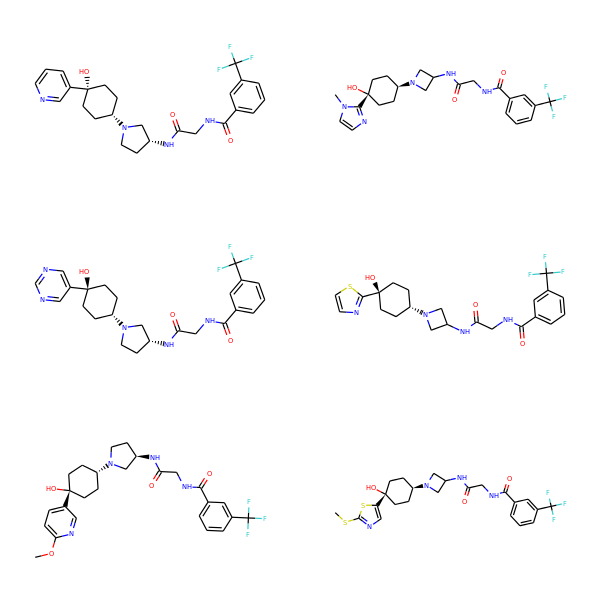# Optimizing cleanup of stereochemistry

A fair amount of the time spent in constructing molecules using the RDKit goes to the assignment/cleanup of stereochemistry. I did an analysis for the 2012 UGM where I found that 27% of the time spent parsing 100K drug-like molecules from the ZINC set was in the assignStereochemistry() function. The function itself is responsible for assigning R/S labels to atoms and, more importantly, removing stereochemistry flags from atoms or double bonds that shouldn't have them (where the substituents are the same).

I think the assignStereochemistry() step is important (to be sure that molecules are actually correctly specified), but it certainly wouldn't be bad to make it faster. That's the point of this exercise.

As a readily availblbe test set for this, I'm going to take the ChEMBL molecules that appeared in documents published betwen 2010 and 2012 that I used in a previous blog post: http://rdkit.blogspot.ch/2013/12/finding-related-documents-in-chembl-2.html. The full set here contains 234681 molecules, since I'm only interested in molecules that have stereochemistry, I narrowed the set down like this:

```egrep '@|/' chembl16_2010-2012.smi > chembl16_2010-2012.chiral.smi
```

That gives 81030 molecules that contain at least one specified chiral center or double bond with specified stereochemistry. For the purposes of this exercise, where I don't want to wait forever, I used the first 40K of those.

As with any optimization exercise, I did this one using the profiler and a relatively simple bit of code that reads in the 40K molecules and then generates canonical smiles for them. The SMILES generation isn't actually important here, but it does provide some useful information about relative timings.

 Operation Percent Time(s) Total 100.0 30.6 Sanitize 26.0 8.0 assignStereochemistry 24.5 7.4 MolToSmiles 39.9 12.2 rankAtoms 21.1 6.4

The rankAtoms step is part of MolToSmiles.

After an embarassing amount of time pursuing avenues that led to no real improvement, I made a couple of small changes (commits https://github.com/rdkit/rdkit/commit/d779c850c9696948f8e718ad790e0224ea7320b8#diff-ca0dbad92a874b2f69b549293387925e and https://github.com/rdkit/rdkit/commit/4d47482f0f9ac7d67be6a811232651da8e5dc635#diff-ca0dbad92a874b2f69b549293387925e) that ended up helping a fair amount:

 Operation Percent Time(s) Total 100.0 28.8 Sanitize 26.9 7.7 assignStereochemistry 20.3 5.8 MolToSmiles 42.9 12.3 rankAtoms 23.8 6.8

The rankAtoms step is part of MolToSmiles.

# Stats about the dataset

• Number of compounds considered: 40000
• Number where stereochemistry was resolved in one pass: 38282
• Number where dependent stereochemistry required two passes: 1712
• Number where dependent stereochemistry required three passes: 6

## Molecules where three passes were required

• CN(C)C(=O)N[C@@H]1CC[C@@H](CN2[C@@H]3CC[C@H]2C[C@H](C3)Oc4cccc(c4)C(=O)N)CC1
• CN(C)C(=O)N[C@@H]1CC[C@@H](CCN2[C@@H]3CC[C@H]2C[C@H](C3)Oc4cccc(c4)C(=O)N)CC1
• CN(C)C(=O)N[C@@H]1CC[C@H](CN2[C@@H]3CC[C@H]2C[C@H](C3)Oc4cccc(c4)C(=O)N)CC1
• CN(C)C(=O)N[C@@H]1CC[C@H](CCN2[C@@H]3CC[C@H]2C[C@H](C3)Oc4cccc(c4)C(=O)N)CC1
• Cc1ccc(s1)C(=CCCN2C[C@@H]3[C@H](C2)[C@@H]3C(=O)O)c4ccc(C)s4
• Cc1ccc(s1)C(=CCCN2C[C@@H]3[C@H](C2)[C@H]3C(=O)O)c4ccc(C)s4

Those all look reasonable.

# More on finding related documents in ChEMBL.

This is a followup to this post, the original notebook is here.

This time I will use Andrew Dalke's very nice, and super fast, chemfp to do the similarity comparisons. This allows much larger data sets to be run, so I'll look at documents from 2010-2012 and, in a second step, use a lower similarity threshold to define neighbors.

In :
```from rdkit import Chem,DataStructs
import time,random
from collections import defaultdict
import psycopg2
from rdkit.Chem import Draw,PandasTools,rdMolDescriptors
from rdkit.Chem.Draw import IPythonConsole
from rdkit import rdBase
from __future__ import print_function
import requests
from xml.etree import ElementTree
import pandas as pd
%load_ext sql
print(rdBase.rdkitVersion)
```
```2014.03.1pre

```

## Preparation

Let's start by getting all molecules from papers from the years 2010-2012 from ChEMBL_16.

In :
```data = %sql postgresql://localhost/chembl_16 \
select molregno,canonical_smiles smiles from \
(select distinct molregno from \
(select doc_id from docs where year>=2010 and year<=2012) t1 \
join (select doc_id,molregno from activities) t2 \
using (doc_id)) tbl \
join compound_structures using (molregno) ;
```
```234681 rows affected.

```
In :
```outf=file('../data/chembl16_2010-2012.smi','w+')
for i,(molregno,smi) in enumerate(data):
outf.write('%s\t%d\n'%(smi,molregno))
outf=None
```
In :
```outf=file('../data/chembl16_2010-2012.mfp2.fps','w+')
outf.write("""#FPS1
#num_bits=2048
#software=RDKit/%s
"""%rdBase.rdkitVersion)

for i,(molregno,smi) in enumerate(data):
mol = Chem.MolFromSmiles(str(smi))
if not mol:
continue
fp = rdMolDescriptors.GetMorganFingerprintAsBitVect(mol,2,nBits=2048)
outf.write("%s\t%d\n"%(DataStructs.BitVectToFPSText(fp),molregno))
if i and not i%(5000):
print("Done: %s"%i)
outf=None
```
```Done: 5000
Done: 10000
Done: 15000
```
In :
```data=None
```
In []:
```t1=time.time()
rows = !simsearch --NxN -t 0.8 ../data/chembl16_2010-2012.mfp2.fps
t2=time.time()
print("That took %.2f seconds"%(t2-t1))
```
In :
```len(rows)
```
Out:
```234594
```

To be clear on exactly how amazing chemfp is: I just found all the pairs in a 230Kx230K set of fingerprints in about two minutes on my three year old desktop linux box.

Limit the data to include only rows for molecules that have at least one neighbor:

In :
```rowsWithData=[x for x in rows if x not in '#0']
len(rowsWithData)
```
Out:
```122744
```
In :
```rowsWithData
```
Out:
```'2\t23\t631370\t1.00000\t705001\t1.00000'
```

Grab doc_ids for each compound:

In :
```data = %sql \
select molregno,doc_id from \
(select doc_id from docs where year>=2010 and year<=2012) t1 \
join (select doc_id,molregno from activities) t2 \
using (doc_id) ;
molDocs=defaultdict(list)
for regno,doc_id in data:
if doc_id not in molDocs[regno]:
molDocs[regno].append(doc_id)
data=None
```
```1362384 rows affected.

```

another data structure to hold the compounds in each document:

In :
```docMols=defaultdict(list)
for regno,docs in molDocs.iteritems():
for docId in docs:
docMols[docId].append(regno)
```

And now figure out the document pairs based on the compound pairs:

In :
```docPairs=defaultdict(list)
molSims={}
for row in rowsWithData:
row = row.split('\t')
nNbrs = int(row)
bRegno = int(row)
for i in range(nNbrs):
iRegno=int(row[2*i+2])
if bRegno==iRegno:
continue
sim=float(row[2*i+3])
if bRegno<iRegno:
tpl=(bRegno,iRegno)
else:
tpl=(iRegno,bRegno)
molSims[tpl]=sim
for dId1 in molDocs[bRegno]:
for dId2 in molDocs[iRegno]:
if dId1==dId2:
continue
if dId1<dId2:
dtpl = (dId1,dId2)
else:
dtpl = (dId2,dId1)
if tpl not in docPairs[dtpl]:
docPairs[dtpl].append(tpl)

```

Put the data into a DataFrame so that it's easier to look at:

In :
```keys=['docid1','docid2','nDoc1','nDoc2','nInCommon','pair_count']
rows=[]
for k,v in docPairs.iteritems():
row=[]
row.append(k)
row.append(k)
s0=set(docMols[k])
s1=set(docMols[k])
row.append(len(s0))
row.append(len(s1))
row.append(len(s0.intersection(s1)))
row.append(len(v))
rows.append(row)
df = pd.DataFrame(data=rows,columns=keys)
```

Add our computed summary properties:

In :
```df['compound_id_tani']=df.apply(lambda row: float(row['nInCommon'])/(row['nDoc1']+row['nDoc2']-row['nInCommon']),axis=1)
df['frac_high_sim']=df.apply(lambda row: float(row['pair_count'])/(row['nDoc1']*row['nDoc2']),axis=1)
```
In :
```df[(df.nDoc1>10)&(df.nDoc2>10)].sort('frac_high_sim',ascending=False).head(10)
```
Out:
docid1 docid2 nDoc1 nDoc2 nInCommon pair_count compound_id_tani frac_high_sim
23277 50562 59446 14 33 0 190 0.000000 0.411255
1332 52860 66739 15 11 1 63 0.040000 0.381818
21840 57757 61248 17 20 4 121 0.121212 0.355882
58593 54004 65337 14 15 2 47 0.074074 0.223810
87061 51349 55319 15 36 15 114 0.416667 0.211111
19563 57623 60600 12 25 11 61 0.423077 0.203333
8744 65426 65439 15 22 1 63 0.027778 0.190909
24548 54604 57777 16 16 2 48 0.066667 0.187500
25254 53089 57738 27 21 2 106 0.043478 0.186949
21699 55412 58519 13 43 11 103 0.244444 0.184258

Take a look at the document titles from pubmed:

In :
```# turn off row count printing
%config SqlMagic.feedback = False

titlePairs=[]
for id_,row in df[(df.nDoc1>10)&(df.nDoc2>10)].sort('frac_high_sim',ascending=False).head(10).iterrows():
did1=row['docid1']
did2=row['docid2']
pmids=%sql  select doc_id,pubmed_id,title from docs where doc_id in (:did1,:did2)
tpl = pmids,pmids
if tpl is None:
print('no pmid found for item: %s'%(str(pmids)))
continue
if tpl is None:
print('no pmid found for item: %s'%(str(pmids)))
continue

txt=requests.get('http://eutils.ncbi.nlm.nih.gov/entrez/eutils/esummary.fcgi?db=pubmed&id=%d,%d'%tpl).text
et = ElementTree.fromstring(txt.encode('utf-8'))
ts=[x.text for x in et.findall(".//*[@Name='Title']")]
titlePairs.append(ts)
print(str(tpl))
print('   '+ts)
print('   '+ts)
```
```(20180534L, 21978682L)
Discovery of a novel series of semisynthetic vancomycin derivatives effective against vancomycin-resistant bacteria.
Synthesis and antibacterial activity of N4-mono alkyl derivatives of novel glycopeptide LYV07ww01.
no pmid found for item: (66739, None, None)
(21601450L, 22244938L)
Novel, potent, and orally bioavailable phosphinic acid inhibitors of the hepatitis C virus NS3 protease.
Discovery of GS-9256: a novel phosphinic acid derived inhibitor of the hepatitis C virus NS3/4A protease with potent clinical activity.
(20951594L, 22989907L)
```

And then inspect the results:

In :
```for id_,row in df[(df.nDoc1>10)&(df.nDoc2>10)].sort('frac_high_sim',ascending=False).head(10).iterrows():
did1=row['docid1']
did2=row['docid2']
pmids=%sql  select doc_id,pubmed_id,chembl_id,year from docs where doc_id in (:did1,:did2)
print('https://www.ebi.ac.uk/chembl/doc/inspect/%s (%d )- https://www.ebi.ac.uk/chembl/doc/inspect/%s (%d)'%(pmids,pmids,pmids,pmids))
```
```https://www.ebi.ac.uk/chembl/doc/inspect/CHEMBL1155426 (2010 )- https://www.ebi.ac.uk/chembl/doc/inspect/CHEMBL1909570 (2011)
https://www.ebi.ac.uk/chembl/doc/inspect/CHEMBL1250472 (2010 )- https://www.ebi.ac.uk/chembl/doc/inspect/CHEMBL2177087 (2012)
https://www.ebi.ac.uk/chembl/doc/inspect/CHEMBL1777720 (2011 )- https://www.ebi.ac.uk/chembl/doc/inspect/CHEMBL1955809 (2012)
https://www.ebi.ac.uk/chembl/doc/inspect/CHEMBL1287679 (2010 )- https://www.ebi.ac.uk/chembl/doc/inspect/CHEMBL2146376 (2012)
https://www.ebi.ac.uk/chembl/doc/inspect/CHEMBL1155521 (2010 )- https://www.ebi.ac.uk/chembl/doc/inspect/CHEMBL1667745 (2011)
https://www.ebi.ac.uk/chembl/doc/inspect/CHEMBL1777703 (2010 )- https://www.ebi.ac.uk/chembl/doc/inspect/CHEMBL1933006 (2011)
https://www.ebi.ac.uk/chembl/doc/inspect/CHEMBL2150961 (2012 )- https://www.ebi.ac.uk/chembl/doc/inspect/CHEMBL2151012 (2012)
```

Once again, many of these don't have ChEMBL matches identified. This is at least partially due to the fact that papers have to cite each other to show up (thanks to George for pointing that out), but another part is clearly going to be the low absolute overlap between papers.

The exception is CHEMBL1255554 - CHEMBL1777727, which is a pair in the current ChEMBL scheme.

# look at some molecules

In :
```d1,d2=50562,59446
mPairs=docPairs[(d1,d2)][:]
random.seed(d1)
random.shuffle(mPairs)
ms = []
for i,j in mPairs[:3]:
d = %sql select m from rdk.mols where molregno=:i
ms.append(Chem.MolFromSmiles(d))
d = %sql select m from rdk.mols where molregno=:j
ms.append(Chem.MolFromSmiles(d))
Draw.MolsToGridImage(ms,molsPerRow=2,subImgSize=(300,300))
```
Out:The molecules do certainly seem similar to each other, and they definitely highlight some nice opportunities for improvement of the depiction code (positive attitude! positive attitude!).

In :
```d1,d2=52860, 66739
mPairs=docPairs[(d1,d2)][:]
random.seed(d1)
random.shuffle(mPairs)
ms = []
for i,j in mPairs[:3]:
d = %sql select m from rdk.mols where molregno=:i
ms.append(Chem.MolFromSmiles(d))
d = %sql select m from rdk.mols where molregno=:j
ms.append(Chem.MolFromSmiles(d))
Draw.MolsToGridImage(ms,molsPerRow=2,subImgSize=(400,200))
```
Out:# Try a lower similarity threshold

In :
```t1=time.time()
rows = !simsearch --NxN -t 0.6 ../data/chembl16_2010-2012.mfp2.fps
t2=time.time()
print("That took %.2f seconds"%(t2-t1))
```
```That took 220.68 seconds

```

It takes a bit longer with the lower threshold, but chemfp is still excellently fast.

In :
```len(rows)
```
Out:
```234594
```
In :
```rowsWithData=[x for x in rows if x not in '#0']
len(rowsWithData)
```
Out:
```216200
```
In :
```data = %sql \
select molregno,doc_id from \
(select doc_id from docs where year>=2010 and year<=2012) t1 \
join (select doc_id,molregno from activities) t2 \
using (doc_id) ;
molDocs=defaultdict(list)
for regno,doc_id in data:
if doc_id not in molDocs[regno]:
molDocs[regno].append(doc_id)
data=None

docMols=defaultdict(list)
for regno,docs in molDocs.iteritems():
for docId in docs:
docMols[docId].append(regno)

docPairs=defaultdict(list)
molSims={}
for row in rowsWithData:
row = row.split('\t')
nNbrs = int(row)
bRegno = int(row)
for i in range(nNbrs):
iRegno=int(row[2*i+2])
if bRegno==iRegno:
continue
sim=float(row[2*i+3])
if bRegno<iRegno:
tpl=(bRegno,iRegno)
else:
tpl=(iRegno,bRegno)
molSims[tpl]=sim
for dId1 in molDocs[bRegno]:
for dId2 in molDocs[iRegno]:
if dId1==dId2:
continue
if dId1<dId2:
dtpl = (dId1,dId2)
else:
dtpl = (dId2,dId1)
if tpl not in docPairs[dtpl]:
docPairs[dtpl].append(tpl)

keys=['docid1','docid2','nDoc1','nDoc2','nInCommon','pair_count']
rows=[]
for k,v in docPairs.iteritems():
row=[]
row.append(k)
row.append(k)
s0=set(docMols[k])
s1=set(docMols[k])
row.append(len(s0))
row.append(len(s1))
row.append(len(s0.intersection(s1)))
row.append(len(v))
rows.append(row)
df = pd.DataFrame(data=rows,columns=keys)

df['compound_id_tani']=df.apply(lambda row: float(row['nInCommon'])/(row['nDoc1']+row['nDoc2']-row['nInCommon']),axis=1)
df['frac_high_sim']=df.apply(lambda row: float(row['pair_count'])/(row['nDoc1']*row['nDoc2']),axis=1)

df[(df.nDoc1>10)&(df.nDoc2>10)].sort('frac_high_sim',ascending=False).head(10)
```
Out:
docid1 docid2 nDoc1 nDoc2 nInCommon pair_count compound_id_tani frac_high_sim
191796 50562 59446 14 33 0 462 0.000000 1.000000
144402 59446 62617 33 19 0 627 0.000000 1.000000
208144 66523 66968 12 27 1 323 0.026316 0.996914
64413 50562 62617 14 19 1 265 0.031250 0.996241
154921 55780 57070 15 15 2 217 0.071429 0.964444
43395 55788 57575 99 37 1 3461 0.007407 0.944854
177126 60278 62777 54 36 2 1817 0.022727 0.934671
194340 61045 66790 26 29 0 700 0.000000 0.928382
233742 51545 53478 30 13 1 341 0.023810 0.874359
68093 65507 66530 21 13 0 236 0.000000 0.864469
In :
```titlePairs=[]
for id_,row in df[(df.nDoc1>10)&(df.nDoc2>10)].sort('frac_high_sim',ascending=False).head(10).iterrows():
did1=row['docid1']
did2=row['docid2']
pmids=%sql  select doc_id,pubmed_id,title from docs where doc_id in (:did1,:did2)
tpl = pmids,pmids
if tpl is None:
print('no pmid found for item: %s'%(str(pmids)))
continue
if tpl is None:
print('no pmid found for item: %s'%(str(pmids)))
continue

txt=requests.get('http://eutils.ncbi.nlm.nih.gov/entrez/eutils/esummary.fcgi?db=pubmed&id=%d,%d'%tpl).text
et = ElementTree.fromstring(txt.encode('utf-8'))
ts=[x.text for x in et.findall(".//*[@Name='Title']")]
titlePairs.append(ts)
print(str(tpl))
print('   '+ts)
print('   '+ts)
```
```(20180534L, 21978682L)
Discovery of a novel series of semisynthetic vancomycin derivatives effective against vancomycin-resistant bacteria.
Synthesis and antibacterial activity of N4-mono alkyl derivatives of novel glycopeptide LYV07ww01.
(21978682L, 22765891L)
Synthesis and antibacterial activity of N4-mono alkyl derivatives of novel glycopeptide LYV07ww01.
Synthesis and antibacterial activity against Clostridium difficile of novel demethylvancomycin derivatives.
(22115594L, 23107481L)
```
In :
```for id_,row in df[(df.nDoc1>10)&(df.nDoc2>10)].sort('frac_high_sim',ascending=False).head(10).iterrows():
did1=row['docid1']
did2=row['docid2']
pmids=%sql  select doc_id,pubmed_id,chembl_id,year from docs where doc_id in (:did1,:did2)
print('https://www.ebi.ac.uk/chembl/doc/inspect/%s (%d )- https://www.ebi.ac.uk/chembl/doc/inspect/%s (%d)'%(pmids,pmids,pmids,pmids))
```
```https://www.ebi.ac.uk/chembl/doc/inspect/CHEMBL1155426 (2010 )- https://www.ebi.ac.uk/chembl/doc/inspect/CHEMBL1909570 (2011)
https://www.ebi.ac.uk/chembl/doc/inspect/CHEMBL1909570 (2011 )- https://www.ebi.ac.uk/chembl/doc/inspect/CHEMBL2057152 (2012)
https://www.ebi.ac.uk/chembl/doc/inspect/CHEMBL2177090 (2012 )- https://www.ebi.ac.uk/chembl/doc/inspect/CHEMBL2203286 (2012)
https://www.ebi.ac.uk/chembl/doc/inspect/CHEMBL1155426 (2010 )- https://www.ebi.ac.uk/chembl/doc/inspect/CHEMBL2057152 (2012)
https://www.ebi.ac.uk/chembl/doc/inspect/CHEMBL1681720 (2011 )- https://www.ebi.ac.uk/chembl/doc/inspect/CHEMBL1759899 (2011)
https://www.ebi.ac.uk/chembl/doc/inspect/CHEMBL1681728 (2011 )- https://www.ebi.ac.uk/chembl/doc/inspect/CHEMBL1772969 (2011)
https://www.ebi.ac.uk/chembl/doc/inspect/CHEMBL1921735 (2011 )- https://www.ebi.ac.uk/chembl/doc/inspect/CHEMBL2062386 (2012)
https://www.ebi.ac.uk/chembl/doc/inspect/CHEMBL1949557 (2011 )- https://www.ebi.ac.uk/chembl/doc/inspect/CHEMBL2203263 (2012)
https://www.ebi.ac.uk/chembl/doc/inspect/CHEMBL1165944 (2010 )- https://www.ebi.ac.uk/chembl/doc/inspect/CHEMBL1268919 (2010)
```
In :
```d1,d2=65507,66530
mPairs=docPairs[(d1,d2)][:]
random.seed(d1)
random.shuffle(mPairs)
ms = []
for i,j in mPairs[:3]:
d = %sql select m from rdk.mols where molregno=:i
ms.append(Chem.MolFromSmiles(d))
d = %sql select m from rdk.mols where molregno=:j
ms.append(Chem.MolFromSmiles(d))
Draw.MolsToGridImage(ms,molsPerRow=2,subImgSize=(300,200))
```
Out:In :
```d1,d2=61045,66790
mPairs=docPairs[(d1,d2)][:]
random.seed(d1)
random.shuffle(mPairs)
ms = []
for i,j in mPairs[:3]:
d = %sql select m from rdk.mols where molregno=:i
ms.append(Chem.MolFromSmiles(d))
d = %sql select m from rdk.mols where molregno=:j
ms.append(Chem.MolFromSmiles(d))
Draw.MolsToGridImage(ms,molsPerRow=2,subImgSize=(300,200))
```
Out:In []: